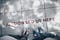Photo by Ian Schneider

# RL — Basics algorithms and terms

Summary of basic Reinforcement learning algorithms, terms & concepts.

# Definitions

Markov Decision Process (MDP)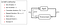Source

Horizon: the number of time steps that we sample or simulate.

Discount factor: discount future rewards.

Notation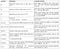Source

Other convention:

Reinforcement learning

Value function

State-value function (total rewards from state s):

Action-value function (total rewards from taking action a in state s)

Advantage functions:

Optimal Value Function V*

Optimal policy

Linear system with additive Gaussian noise.

Linear Gaussian controller

Linear Gaussian dynamics

# Reinforcement Algorithms

Value Iterations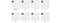Source

Bellman equation:

Algorithms: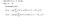Source

Q-value iterations

Bellman equation:

Iteration:

Policy iterations

Policy evaluation

Iterations: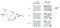Source

Algorithm: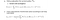Source

Q-learning (SARSA Max)

Algorithm: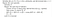Source

Deep Q-learning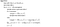Source

Policy Gradient

Monte-Carlo Policy Gradient with a baseline: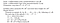Source

Natural Policy Gradient

TRPO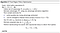Source

Backtracking line search:

Actor-critic algorithm

One-step Actor-critic

A3C

A3C is the asynchronous version of A2C.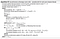Source

DDPG

Adding parameter noise for exploration: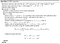Source

Monte Carlo Tree Search (MCTS)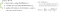Modified from source

Importance sampling

In RL, we may want to estimates the value functions for a policy π but we only collect sample results from π’. Importance sampling allows us to evaluate a policy from another policy.

Importance sampling is a technique of estimating the expected value of f(x) where x has the data distribution p. However, Instead of sampling from p, we calculate the result from sampling q.

We sample data from q and estimate the expected value for p using:

For this to work q(xi) cannot be zero if the corresponding p(xi) is not zero. Let’s demonstrate it with an example and compute 𝔼[f(x)] with data distribution x1 and x2 separately.

Now, we re-estimate the expected value for x2 from sampling results using x1.

In RL, we sample results using the current policy π1 and we can use importance sampling to estimate the result if we change to policy π2.

Advantage function with importance sampling.

Advantage function is the discounted rewards minus a baseline. There are many possible ways to compute value functions (discounted rewards) including Monte Carlo rollout, temporal difference, or a combination of both. For completeness, the following is a k-step look ahead used in A3C to compute the advantage function. This is a k-step rollout. We observe the rewards and baseline it with the function value of the corresponding state which can be approximated by a deep network.Source

There are many possible baselines. Different baselines can reach the same optimal point as long as it is not depend on the policy model θ. So based on the choice of computing the value functions, you can select the corresponding baseline to reduce the variance of the calculation.

Importance sampling calculates the Advantage function A by sampling Â using the current policy θk. Then the result is recalibrated for the new policy θ using the probability ratio between these two policies.

Trust region method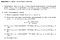Source

ε-greedy policy

To sample the kth episode, we use a ε-greedy algorithm below to pick which action to sample next. Here is the data distribution used in selecting the action:

Dyna-Q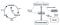Source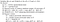Source

DAgger: Dataset Aggregation

DAgger is an algorithm to argument the training dataset with expert demonstrations for states that may visited by the current learned policy. So even if the current policy is in-accurate or drifted, we can take corrective actions.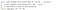Source

# Optimization algorithm

Newton’s method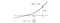Source

Solving f(x)=0:

Minimize f(x):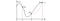Source

Nonlinear least-squares problem with Gauss-Newton method

Newton’s method on optimization:

According to the equation above, the search direction p (a.k.a. Δx), using Newton’s method, can be written as:

Compute the first and second order derivative of f:

In the Gauss-Newton method, we approximate the second derivative above without the Q term. The Q term is smaller than the first term and if the problem has zero residual, r = Q = 0. Gauss-Newton method applies this approximation to the Newton’s method.

The Gauss-Newton method search direction is:

Let’s have an example, we want to determine the growth rate of antelope. We develop a model g and fit data into the the model to compute the residual error r: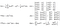Source

Conjugate gradient

We use conjugate gradient method to optimize a quadratic equation or to solve a linear equation.

In a line search, we determine the direction of ascent and then select the step size. For example, in the gradient ascent, we follow the direction with the steepest gradient and take a step with size equal to the gradient times the learning rate. In the example below, the deepest direction, according to the gradient contour, is to move right. The next move may go up and a little bit to the left. Even we are getting closer to the optimal point in every move, we may ask why every move undo part of the previous move (for example, going a little bit left in the second move)

The conjugate gradient method is a line search method with careful choice of coordinated directions. It optimizes a quadratic equation in much fewer step than the gradient ascent. If x is N-dimensional (N parameters), we can find the optimal point in at most n steps. For every next move, we want a direction conjugate to all previous moves. Mathematically, it means any new direction dj must obey the following with any previous direction di:

where A is the matrix in the quadratic equation.

We can visualize this in our example as moving perpendicular to the previous ones.

This is the algorithm. We start with a random (or educated) guess Xo and use the equation below to calculate where should we go next X1. p is the direction to go (equivalent to d above)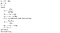wikipedia

Let’s define two terms:

• e is the error between the current guess and the optimal point.
• r measures how far we are from the correct value b. We can view that as the error e transformed by A to the space of b.

From,

We can derive:

Say our next point is computed as:

In order for the future direction not to undo progress from the previous directions, let’s try d and e to be orthogonal:

α depends on e which we don’t know its value. So instead of orthogonal, let’s try all searching direction d to be A-orthogonal:

To fulling these conditions, we will move xi in the direction d to the optimal point in the search direction d.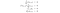Source

Using the A-orthogonal requirement, α is computed as: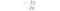Source

So conjugate gradient is about finding an A-orthogonal search direction every time and move to the optimal point in that search direction. These vectors are independent of each other and span a N-dimensional space. So we can solve in at most n steps.

# Terms

Taylor series

In vector form:

where g is the Jacobian matrix and H is the Hessian matrix.

Jacobian matrix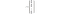Jacobian matrix

Hessian matrix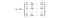Hessian matrix

Examples: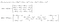Examples from source

KL-divergence

In deep learning, we want a model predicting data distribution Q resembling the distribution P from the data. Such difference between two probability distributions can be measured by KL Divergence which is defined as:

Positive-definite matrix

Matrix A is positive-definite if

for all real non-zero vector z.

# Concepts

Supervised learning v.s. unsupervised learning v.s. reinforcement learning

Supervised learning is learning from a training dataset provided with labels from knowledgable sources (e.g. the human). For example, we want to learn the object class (label) of an object inside a picture (a data sample). When the system is presented with a new sample, it should generalize what it learns to answer that question “what is the label?”.

Unsupervised learning explores the hidden structure of the data. For example, what is the latent features of the data sample or what category (cluster) the data belong to.

Reinforcement learning observers the environment and takes actions to maximize the rewards (goals). It mainly deals with exploration, exploitation, trial-and-error search, delayed rewards, system dynamics (how the environment reacts to the actions taken) and defining goals.

Agent (Controller)

The system (like robots) that interacts and acts on the environment.

Policy

A policy defines how the learning agent may act from a specific state. Mathematically, it is the probability of taking an action a given the state s. π = p(a | s)

Rewards

A reward signal (r(s, a, s’)) defines the goal in a reinforcement learning problem. For example, that is the score in a game or turning a door knob for a robotic arm. The total rewards is the return.

Value function (state-value function)

The value of a state is the total amount of rewards that an agent can collect from now to the end V(s).

Action-value function

The value of taking an action from a state is the total amount of rewards that an agent can collect from now to the end by taken a specific action Q(s, a).

Model of the environment

A model describe how an environment may change upon an action from a state p(s, a, s’).

Episodes

An episode plays through the whole sequence (e.g. a game) until reaching the terminal state.

Discount rate γ

Discount rate values the future rewards at the present value.

Model v.s. model free

In model free RL, the system dynamic is unknown or not needed.

Monte Carlo Method

Monte Carlo methods play through a completed episode. It computes the average of the sample returns from multiple episodes to estimate value functions. The following creates a running average using the Monte Carlo method.

Monte Carlo control

Use Monte Carlo methods to evaluate the current policy. Using the estimated value functions, we improve the current policy.

On-policy learning v.s. off-policy learning

We use a policy to determine which actions to take. In on-policy learning, we optimize the current policy and use it to determine what actions to explore next. Since the current policy is not optimized or sub-optimized, it still allows some form of exploration. Off-policy learning allows a second policy, like the ε-greedy policy, to explicitly define the exploration it wants. Off-policy learning optimizes the current policy but use another policy to explore actions. Off-policy provides greater choice but slower to converge and have higher complexity. Off-policy learning mostly utilizes importance sampling in evaluating policy.

Prediction

Policy evaluation

Temporal-Difference Learning (TD)

Instead of completing the whole episode like the Monte Carlo:

We rollout k-steps and collect the rewards. We estimate the value function based on the collected rewards and the value function after k-steps. Below is an 1-step TD learning. We find our the reward after taking one action and find out the value function for the new state.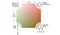Source

Planning

We use the system dynamics (model) to generate simulated experience and use them to refit the value functions or the policy.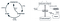Source

The difference between learning and planning is one from real experience generated by the environment and one from simulated experience by a model.

The following is the Dyna-Q algorithm which it uses the learned model to refit the Q-function values.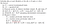Source

Trajectory optimization

Shooting methods

Optimize trajectory based on an open-loop system. Observe the initial (first) state & optimize the corresponding actions.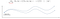Source

For a stochastic system, this is suboptimal because we do not readjust the actions based on the observed states where we transit to.

Collocation methods

Optimize trajectory based on a closed-loop system which we take actions based on the observed states.

Welcome to a place where words matter. On Medium, smart voices and original ideas take center stage - with no ads in sight. Watch
Follow all the topics you care about, and we’ll deliver the best stories for you to your homepage and inbox. Explore
Get unlimited access to the best stories on Medium — and support writers while you’re at it. Just \$5/month. Upgrade